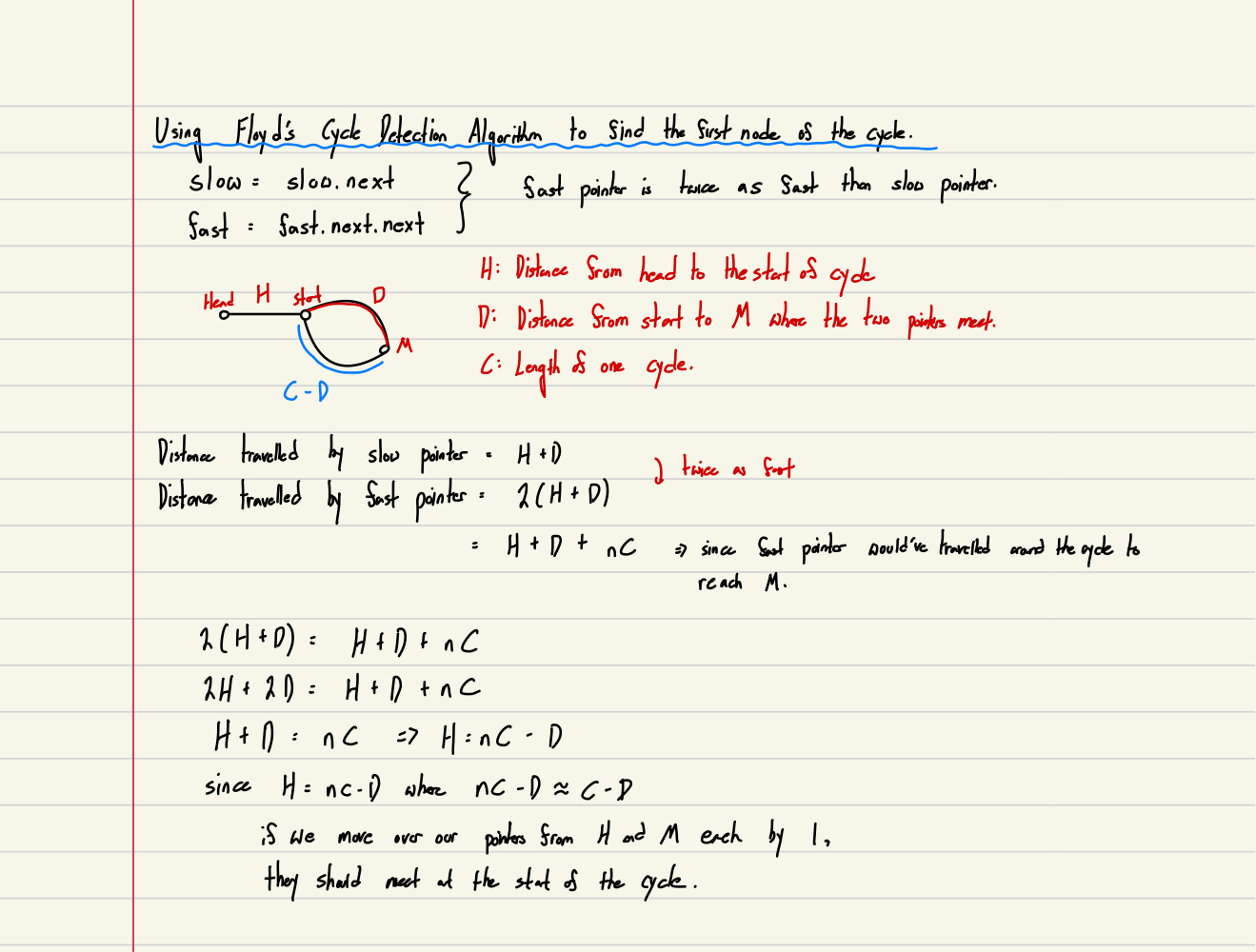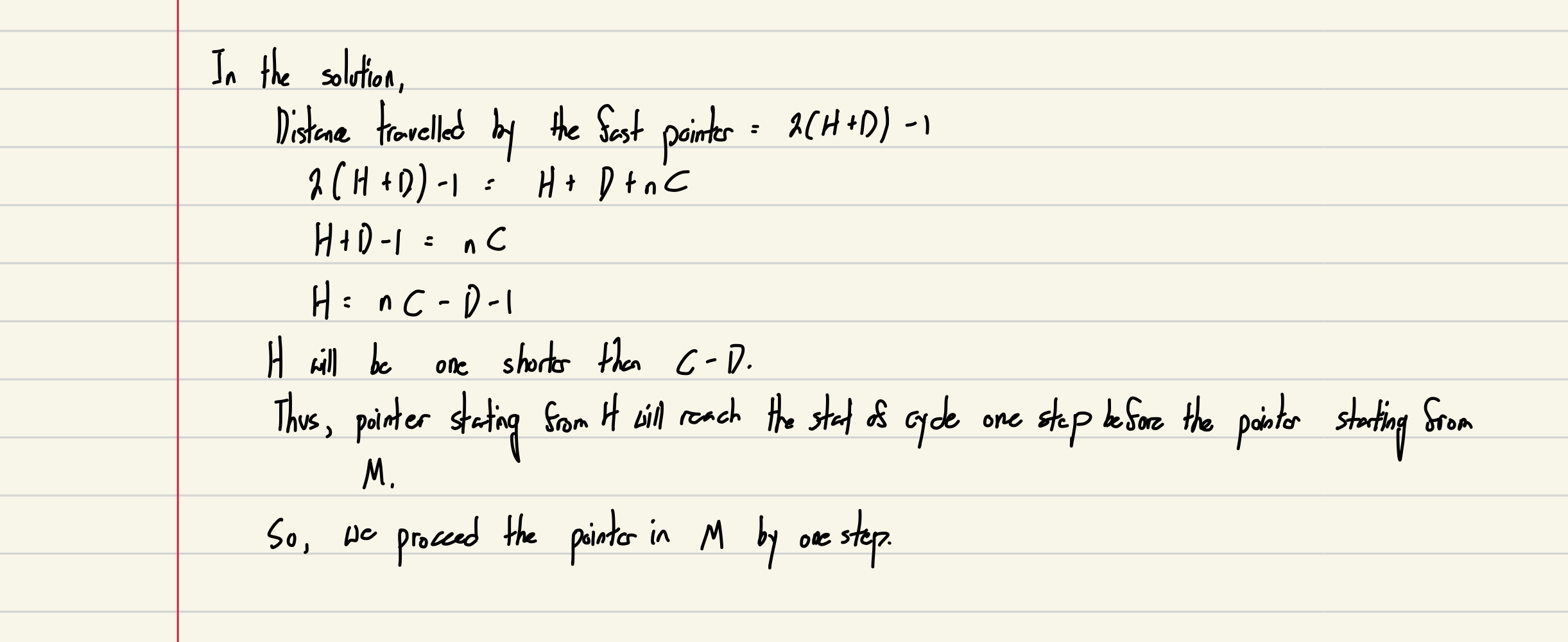# [LeetCode] #142: Linked List Cycle II

choikh0423·2022년 5월 17일
0

## LeetCode

목록 보기
11/11## Problem:

Given the head of a linked list, return the node where the cycle begins. If there is no cycle, return null.

There is a cycle in a linked list if there is some node in the list that can be reached again by continuously following the next pointer. Internally, pos is used to denote the index of the node that tail's next pointer is connected to (0-indexed). It is -1 if there is no cycle. Note that pos is not passed as a parameter.

## Process:

Objective: Return the node where the cycle begins

Constraints:
1. The number of the nodes in the list is in the range $[0, 10^4]$.
2. $-10^5 <= Node.val <= 10^5$
3. pos is -1 or a valid index in the linked-list.

Edge Cases:
1. 0 nodes
2. 1 node
3. more than 1 node

## Brainstorm:

What is a cycle?
Cycle is a repetition. The values in the cycle will be repeated over and over again in that same order.
The tail will be directed towards head again.

It also means that if there are two different pointers running through the list at a different rate, they will meet some time. With this, we can find whether or not the list has a cycle in it.

How do we know where the cycle starts?
The same pattern will occur over and over again starting from the same point. If we could figure out which part of the list is not a reoccuring part of it, the part that follows the non-recurring part would be the start of the cycle.

## Solution

### Attempt #1: Returning the first node that we revisit

• Time Complexity: O(n)
• Space Complexity: O(n)

How it works
The key of this problem is to check at the node object itself, not the value of the node. The value of the node may be repetitive.
1. As we iterate through the list, we store each node in list_tracker.
2. If the node is revisited, we return the node right away.

def detectCycle(self, head: Optional[ListNode]) -> Optional[ListNode]:

list_tracker = set()

while node:
if node in list_tracker:
return node

node = node.next

return None

Limitation:
1. It uses too much space.

### Attempt #2: Applying Floyd's Cycle Detection Algorithm to find the first node

• Time Complexity: O(n)
• Space Complexity: O(1)

How it works:def detectCycle(self, head: Optional[ListNode]) -> Optional[ListNode]:

try:

while slow is not fast:
fast = fast.next.next
slow = slow.next
except:
return None

return head